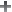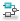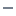## LQR/LQG Goal

### Purpose

Minimize or limit Linear-Quadratic-Gaussian (LQG) cost in response to white-noise inputs, when using Control System Tuner.

### Description

LQR/LQG Goal specifies a tuning requirement for quantifying control performance as an LQG cost. It is applicable to any control structure, not just the classical observer structure of optimal LQG control.

The LQG cost is given by:

J = E(z(t)′ QZ z(t)).

z(t) is the system response to a white noise input vector w(t). The covariance of w(t) is given by:

E(w(t)w(t)′) = QW.

The vector w(t) typically consists of external inputs to the system such as noise, disturbances, or command. The vector z(t) includes all the system variables that characterize performance, such as control signals, system states, and outputs. E(x) denotes the expected value of the stochastic variable x.

The cost function J can also be written as an average over time:

`$J=\underset{T\to \infty }{\mathrm{lim}}E\left(\frac{1}{T}{\int }_{0}^{T}z\left(t\right)\text{'}\text{\hspace{0.17em}}QZ\text{\hspace{0.17em}}z\left(t\right)dt\right).$`

#### Creation

In the Tuning tab of Control System Tuner, select New Goal > LQR/LQG objective to create an LQR/LQG Goal.

#### Command-Line Equivalent

When tuning control systems at the command line, use `TuningGoal.LQG` to specify an LQR/LQG goal.

### Signal Selection

Use this section of the dialog box to specify noise input locations and performance output locations. Also specify any locations at which to open loops for evaluating the tuning goal.

• Specify noise inputs (w)

Select one or more signal locations in your model as noise inputs. To constrain a SISO response, select a single-valued input signal. For example, to constrain the LQG cost for a noise input `'u'` and performance output `'y'`, clickAdd signal to list and select `'u'`. To constrain the LQG cost for a MIMO response, select multiple signals or a vector-valued signal.

• Specify performance outputs (z)

Select one or more signal locations in your model as performance outputs. To constrain a SISO response, select a single-valued output signal. For example, to constrain the LQG cost for a noise input `'u'` and performance output `'y'`, clickAdd signal to list and select `'y'`. To constrain the LQG cost for a MIMO response, select multiple signals or a vector-valued signal.

• Evaluate LQG objective with the following loops open

Select one or more signal locations in your model at which to open a feedback loop for the purpose of evaluating this tuning goal. The tuning goal is evaluated against the open-loop configuration created by opening feedback loops at the locations you identify. For example, to evaluate the tuning goal with an opening at a location named `'x'`, clickAdd signal to list and select `'x'`.

Tip

To highlight any selected signal in the Simulink® model, click. To remove a signal from the input or output list, click. When you have selected multiple signals, you can reorder them usingand. For more information on how to specify signal locations for a tuning goal, see Specify Goals for Interactive Tuning.

### LQG Objective

Use this section of the dialog box to specify the noise covariance and performance weights for the LQG goal.

• Performance weight Qz

Performance weights, specified as a scalar or a matrix. Use a scalar value to specify a multiple of the identity matrix. Otherwise specify a symmetric nonnegative definite matrix. Use a diagonal matrix to independently scale or penalize the contribution of each variable in z.

The performance weights contribute to the cost function according to:

J = E(z(t)′ Qz z(t)).

When you use the LQG goal as a hard goal, the software tries to drive the cost function J < 1. When you use it as a soft goal, the cost function J is minimized subject to any hard goals and its value is contributed to the overall objective function. Therefore, select Qz values to properly scale the cost function so that driving it below 1 or minimizing it yields the performance you require.

• Noise Covariance Qw

Covariance of the white noise input vector w(t), specified as a scalar or a matrix. Use a scalar value to specify a multiple of the identity matrix. Otherwise specify a symmetric nonnegative definite matrix with as many rows as there are entries in the vector w(t). A diagonal matrix means the entries of w(t) are uncorrelated.

The covariance of w(t is given by:

E(w(t)w(t)′) = QW.

When you are tuning a control system in discrete time, the LQG goal assumes:

E(w[k]w[k]′) = QW/Ts.

Ts is the model sample time. This assumption ensures consistent results with tuning in the continuous-time domain. In this assumption, w[k] is discrete-time noise obtained by sampling continuous white noise w(t) with covariance QW. If in your system w[k] is a truly discrete process with known covariance QWd, use the value Ts*QWd for the QW value.

### Options

Use this section of the dialog box to specify additional characteristics of the LQG goal.

• Apply goal to

Use this option when tuning multiple models at once, such as an array of models obtained by linearizing a Simulink model at different operating points or block-parameter values. By default, active tuning goals are enforced for all models. To enforce a tuning requirement for a subset of models in an array, select Only Models. Then, enter the array indices of the models for which the goal is enforced. For example, suppose you want to apply the tuning goal to the second, third, and fourth models in a model array. To restrict enforcement of the requirement, enter `2:4` in the Only Models text box.

### Tips

When you use this requirement to tune a control system, Control System Tuner attempts to enforce zero feedthrough (D = 0) on the transfer that the requirement constrains. Zero feedthrough is imposed because the H2 norm, and therefore the value of the tuning goal, is infinite for continuous-time systems with nonzero feedthrough.

Control System Tuner enforces zero feedthrough by fixing to zero all tunable parameters that contribute to the feedthrough term. Control System Tuner returns an error when fixing these tunable parameters is insufficient to enforce zero feedthrough. In such cases, you must modify the requirement or the control structure, or manually fix some tunable parameters of your system to values that eliminate the feedthrough term.

When the constrained transfer function has several tunable blocks in series, the software’s approach of zeroing all parameters that contribute to the overall feedthrough might be conservative. In that case, it is sufficient to zero the feedthrough term of one of the blocks. If you want to control which block has feedthrough fixed to zero, you can manually fix the feedthrough of the tuned block of your choice.

To fix parameters of tunable blocks to specified values, see View and Change Block Parameterization in Control System Tuner.

### Algorithms

When you tune a control system, the software converts each tuning goal into a normalized scalar value f(x). Here, x is the vector of free (tunable) parameters in the control system. The software then adjusts the parameter values to minimize f(x) or to drive f(x) below 1 if the tuning goal is a hard constraint.

For LQR/LQG Goal, f(x) is given by the cost function J:

J = E(z(t)′ Qz z(t)).

When you use the LQG requirement as a hard goal, the software tries to drive the cost function J < 1. When you use it as a soft goal, the cost function J is minimized subject to any hard goals and its value is contributed to the overall objective function. Therefore, select `Qz` values to properly scale the cost function so that driving it below 1 or minimizing it yields the performance you require.

## Related Topics